Differentiation (Beginning Calculus)

Author:
Lew W. S.
Topic:
Calculus
Basic Differentiation, Chain Rule, Product and Quotient Rules. Applets for drill and practice and for self learning.
•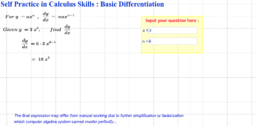Basic Differentiation Self Practice Sheet

•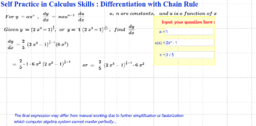Differentiation with Chain Rule Self Practice Sheet

•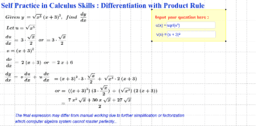Differentiation using Product Rule Self Practice Sheet

•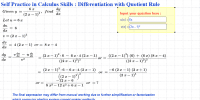Differentiation using Quotient Rule Self Practice Sheet

•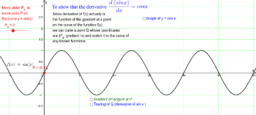First Derivative of Sine x Demonstrated by Graphical Method

•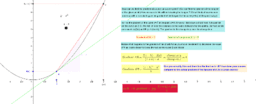Derivative from first principles

•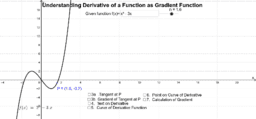Derivative of a Function, and its Gradient Function

•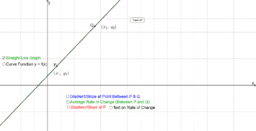Gradient, Average and Instantaneous Rate of Change

•Integral calculation for beginners

•Problems with graphs of first derivatives

•